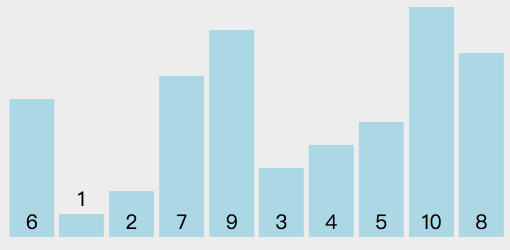## # 思想

• 选择一个基准元素`target`（一般选择第一个数）
• 将比`target`小的元素移动到数组左边，比`target`大的元素移动到数组右边
• 分别对`target`左侧和右侧的元素进行快速排序## # 写法1

``````    function quickSort(array) {
if (array.length < 2) {
return array;
}
const target = array;
const left = [];
const right = [];
for (let i = 1; i < array.length; i++) {
if (array[i] < target) {
left.push(array[i]);
} else {
right.push(array[i]);
}
}
return quickSort(left).concat([target], quickSort(right));
}
``````

## # 写法2

`l<r`的条件下，找到右侧小于`target`的值`array[r]`，并将其赋值到`array[l]`

`l<r`的条件下，找到左侧大于`target`的值`array[l]`，并将其赋值到`array[r]`

``````    function quickSort(array, start, end) {
if (end - start < 1) {
return;
}
const target = array[start];
let l = start;
let r = end;
while (l < r) {
while (l < r && array[r] >= target) {
r--;
}
array[l] = array[r];
while (l < r && array[l] < target) {
l++;
}
array[r] = array[l];
}
array[l] = target;
quickSort(array, start, l - 1);
quickSort(array, l + 1, end);
return array;
}
``````